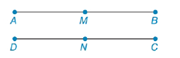Chapter 1.7, Problem 32E### Elementary Geometry for College St...

6th Edition
Daniel C. Alexander + 1 other
ISBN: 9781285195698

#### Solutions

Chapter
Section### Elementary Geometry for College St...

6th Edition
Daniel C. Alexander + 1 other
ISBN: 9781285195698
Textbook Problem
1 views

# In Exercises 27 to 35, complete the formal proof of each theorem.If two line segments are congruent, then their midpoints separate these segments into four congruent segments. Given: A B ¯ ≅ D C ¯ M is midpoint of A B ¯ N is midpoint of D C ¯ Prove: A M ¯ ≅ M B ¯ ≅ D N ¯ ≅ N C ¯To determine

To find:

The complete formal proof of the given theorem.

If two line segments are congruent, then their midpoints separate these segments into four congruent segments.

Explanation

Given:

The statement of given theorem is,

If two line segments are congruent, then their midpoints separate these segments into four congruent segments.

Given: AB¯DC¯, M is midpoint of AB¯ and N is midpoint of DC¯

Prove: AM¯MB¯DN¯NC¯

The given figure is,

Approach:

The formal proof of given theorem is shown in the following table.

 Statements Reasons 1. M is midpoint of AB¯ and N is midpoint of DC¯ 1. Given 2. AM¯≅MB¯ and DN¯≅NC¯ 2. If a midpoint separates the line segment into two parts, then two parts are congruent. 3. AM=MB and DN=NC 3. The length of two congruent line segments is same. 4. AB=AM+MB and DC=DN+NC 4

### Still sussing out bartleby?

Check out a sample textbook solution.

See a sample solution

#### The Solution to Your Study Problems

Bartleby provides explanations to thousands of textbook problems written by our experts, many with advanced degrees!

Get Started

#### Find the median for the following set of scores: 1, 9, 3, 6, 4, 3, 11, 10

Essentials of Statistics for The Behavioral Sciences (MindTap Course List)

#### Evaluate the integrals in Problems 7-36. Check your results by differentiation. 18.

Mathematical Applications for the Management, Life, and Social Sciences

#### Evaluate the integral. 45. x5ex3dx

Single Variable Calculus: Early Transcendentals

#### The directrix of the conic given by is:

Study Guide for Stewart's Multivariable Calculus, 8th

#### True or False: is monotonic.

Study Guide for Stewart's Single Variable Calculus: Early Transcendentals, 8th